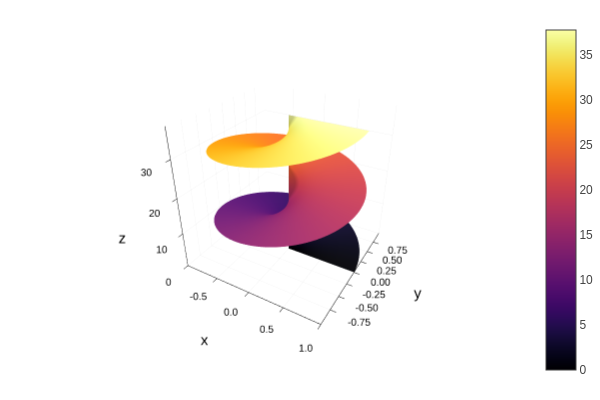# Parametric surface plot of custom indexed array

I am struggling with something which seems pretty trivial problem. Namely, I have a stochastic process on a random graph depending on the two parameters. The first parameter is the probability of an edge between two nodes of a random graph. The second parameter is the number of nodes in a certain state at the beginning of the process. The output is an integer function depending on those two parameters. I would like to get a surface plot

surface(p_edge, num_ian, my_function(p_edge, num_ian))

The following is really naive code which avoids custom indices but I am not 100 sure that p_edge and num_ian are mapped with the correct valued of the function. Ideally, I would like to iterate a 2D array over two directed linearly ordered sets instead of essentially introducing auxiliary variables to avoid custom index sets.

``````#!/usr/bin/julia
using Debugger
using Graphs
using SparseArrays
using StatsBase
using Statistics
using Plots;plotlyjs()

include("my_function.jl")

n = 200
p_edge = 0.01:0.01:0.5
num_ian = 10:1:100

target_surf = Matrix{Int64}(undef, size(p_edge,1), size(num_ian,1))

for i = 1:size(p_edge,1)
g = erdos_renyi(n, p_edge[i]; is_directed=true, seed=-1)
for j = 1:size(num_ian,1)
ia = sample(1:n,num_ian[j]; replace=false, ordered=true)
target_surf[i,j] = my_function(g,ia)
end
end
display(target_surf)
plot(p_edge, num_ian, target_surf, st = :surface, xlabel = "p_edge", ylabel = "num_ian", zlabel = "mine > 1")
``````

Due to my low trust level I can’t edit the post so I am replying to my own question. The above problem can be trivially seen as a parametric surface plot. What I was looking for is the equivalent of Mathematica’s ParametricPlot3D function.

It is definitely doable using vanilla Plots package but the documentation is a bit lacking and it is definitely
not a one-liner. I stumbled onto the package
Axl that is supposedly doing what I want but I don’t want to turn my Julia installation into a package kitchen-sink.

This is a minimal working example of the helicoid plot

``````#!/usr/bin/julia
using Debugger
using Plots;plotlyjs()

X(r,t) = r*cos(t)
Y(r,t) = r*sin(t)
Z(r,t) = 3*t

r_range = LinRange(0, 1, 200)
t_range = LinRange(0, 4*pi, 400)

x_grid = [X(r,t) for r in r_range, t in t_range]
y_grid = [Y(r,t) for r in r_range, t in t_range]
z_grid = [Z(r,t) for r in r_range, t in t_range]

plot(x_grid, y_grid, z_grid, st = :surface, xlabel = "x", ylabel = "y", zlabel = "z")
``````

And the output1 Like